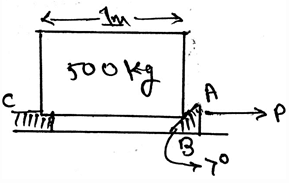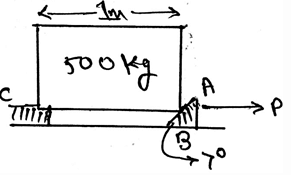# Test: Wedges

## 15 Questions MCQ Test Engineering Mechanics | Test: Wedges

Description
Attempt Test: Wedges | 15 questions in 30 minutes | Mock test for Mechanical Engineering preparation | Free important questions MCQ to study Engineering Mechanics for Mechanical Engineering Exam | Download free PDF with solutions
QUESTION: 1

### A ____________ is a simple machine that is used as to transfer applied forces into much larger forces.

Solution:

The main thing about the wedges is that the wedge is a simple machine that is used as to transfer applied forces into much larger forces. This means that the wedges are the means of force transfer. This machine is very useful in the industries, and it has a wide application.

QUESTION: 2

### Wedges are used to transfer heavy loads.

Solution:

The main thing about the wedges is that the wedge is a simple machine that is used as to transfer applied forces into much larger forces. This means that the wedges are the means of force transfer. This machine is very useful in the industries, and it has a wide application.

QUESTION: 3

### The blocks of heavy weights are being able to lift from the help of the wedges.

Solution:

The main thing about the wedges is that the wedge is a simple machine that is used as to transfer applied forces into much larger forces. This means that the wedges are the means of force transfer. This machine is very useful in the industries, and it has a wide application.

QUESTION: 4

The normal force exerted by the surface of the wedge is normal to the surface of the ________

Solution:

As we know the normal forces act perpendicular to the surfaces of the body kept over the wedges. Thus the normal force exerted by the surface of the wedge is normal to the surface of the base of the body kept over the wedge. The wedges are very useful in the industries, and it has a wide application.

QUESTION: 5

Which one is not the condition for the equilibrium in free body diagram for calculation of the normal forces along the body kept over the wedge, consider all forces to be straight and linear?

Solution:

For the equilibrium in the three dimensional system of axis we have all the conditions true as, ∑Fx=0, ∑Fy=0 and ∑Fz=0. Also we have the summation of the forces equal to zero. Which is not a non-zero value.

QUESTION: 6

The coefficient of friction between the body being slided over the wedge and the wedge surface is generally determined by ____________

Solution:

The coefficient of the friction is generally determined by the help of experiments. Many experiments are done on the body. Try and error methods are involved. And the final observations are being taken out. Then average of all the final answers resulted in the experiments is done.

QUESTION: 7

When does the two wedge system is termed as self-locking system?

Solution:

If friction forces hold the block in plane the two wedge system is termed as self-locking system. This is generally the concept used to lift up the boxes and then it is also used as to make the very heavy works easy. Thus the wedges are very useful in the industries, and it has a wide application.

QUESTION: 8

Determine the normal force at B in the wedge shown. Take the value of coefficient of friction to be 0.3. Assume that the block doesn’t slip at C.Solution:

The normal force at A is 2381 N. The normal force at B is 2452.5 N. The angle of the wedge over which the block is being slided is generally taken out by the help of the tangent inverse trigonometric function. Thus the answer.

QUESTION: 9

In the free body diagrams involving the wedges we have use of vector math, so for two vectors A and B, what is A.B (if they have angle α between them)?

Solution:

The dot product of the two vectors is always the product of the magnitudes of the two forces and the cosine of the angle between them. We need to consider the triangle and then accordingly apply the trigonometry. This is one of the way of resolving the components.

QUESTION: 10

What is j.j?

Solution:

As the dot product of only the same Cartesian component is unity, i.e. i.i = 1 and j.j =1, rest all remaining dot product will give 0(i.j = 0 and j.k = 0). Cross product of the same plane vectors always give zero. And dot product of the same plane vector gives a scalar quantity.

QUESTION: 11

Choose the correct one.

Solution:

The coplanar system means the consideration is with the 2D only. No 3D. Thus if there is any moment needed to be considered we need to take it along the point and not along the axis. This is the concept used in the solving of the problems related to wedges.

QUESTION: 12

Sometimes in the calculations involving the wedges, there are situations when there is a toppling effect on the body. In that if the net moment of the body is zero that means the distance between the force and the rotational axis is zero.

Solution:

The net moment of the body is zero that doesn’t means that the distance between the force and the rotational axis is zero. This means moments caused by different forces cancels out. If this happens there is no motion of the body along any direction and hence the body is said to be in equilibrium.

QUESTION: 13

The angle of the inclination of wedge over which the block is sliding is determined by which of the following trigonometric function?

Solution:

The angle of the wedge over which the block is being slided is generally taken out by the help of the tangent inverse trigonometric function. It is the ratio of the frictional force to the normal force. This ratio is kept inside the inverse function.

QUESTION: 14

In the simplification of the forces applied in the wedges net force acts at the ___________ of the loading body.

Solution:Use Code STAYHOME200 and get INR 200 additional OFF Use Coupon Code